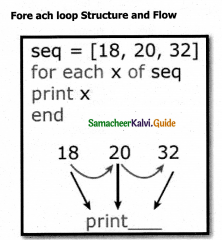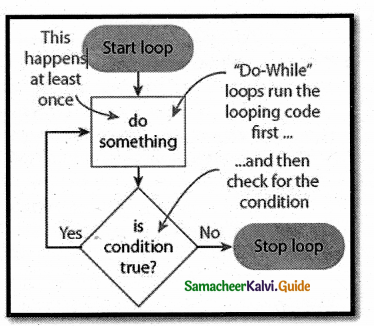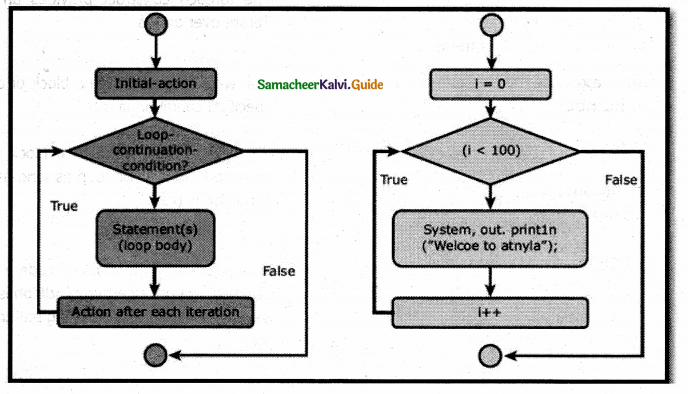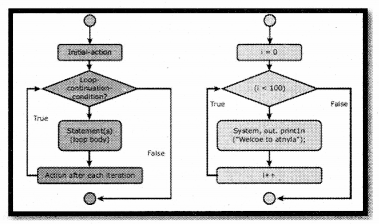Tamilnadu State Board New Syllabus Samacheer Kalvi 12th Computer Applications Guide Pdf Chapter 7 Looping Structure Text Book Back Questions and Answers, Notes.

## Tamilnadu Samacheer Kalvi 12th Computer Applications Solutions Chapter 7 Looping Structure

### 12th Computer Applications Guide Looping Structure Text Book Questions and Answers

Part I

Question 1.
Most complicated looping structure is
a) While
b) Do While
c) For
d) None of them
c) ForQuestion 2.
Loops that iterate for fixed number of times is called
a) Unbounded loops
b) Bounded loops
c) While loops
d) For loops
b) Bounded loops

Question 3.
Which loop evaluates condition expression as Boolean, if it is true, it cutes statements and when it is false it will terminate?
a) For loop
b) For each loop
c) While loop
d) All of them
d) All of them

Question 4.
Which loop evaluates condition expression as Boolean, if it is true, it executes statements and when it is false it will ter-minate?
a) For loop
b) For each loop
c) While loop
d) All of them
d) All of themQuestion 5.
What will be displayed in a browser when the following PHP code is executed;
<?php
for ($counter = 20;$counter < 10;$counter++) { echo “Welcome to Tamilnadu “; } echo “Counter is:$counter”;
?>
b) Counter is: 20
c) Welcome to Tamilnadu counter is: 22
e) Infinite loop
e) Infinite loop

Question 6.
What will be displayed in a browser when the following PHP code is executed;
<?php
for ($counter = 10;$counter < 10; $counter =$counter + 5){ echo “Hello”;
>
?>
a) Hello Hello Hello Hello Hello
b) Hello Hello Hello
c) Hello
d) None of the above
d) None of the aboveQuestion 7.
PHP supports four types of looping techniques;
a) for loop
b) while loop
c) foreach loop
d) all the above
d) all the above

Question 8.
Consider the following code
<? php
$count=12; do{ printf(“%d squared=%d<br/>”,$count,
pow($count,2)); } while($count<4);
?>
What will be the output of the code.

a) 12 squared 141
b) 12 squared=141
c) “12 squared=141
d) Execution error
d) Execution error

Question 9.
What will be the output of the following PHP code ?
<?php
for ($x = 1;$x < 10;++$x) { print “*\t”; } ?> a) ********** b) ********* c) *********** d) infinite l00p Answer: b) *********Question 10. What will be the output of the following PH P code? <?php for ($x = -1; $x < 10;–$x)
{
print $x; } ?> a)123456713910412 b)123456713910 c) 1234567139104 d) Infinite loop Answer: d) Infinite loop Part II Short Answers Question 1. Define Looping Structure in PHP. Answer: 1. Looping Structures are useful for writing iteration logics. 2. It is the most important feature of many programming languages, including PHP. 3. They are implemented using the following categories, • for Loop • While Loop • foreach Loop • Do While Loop Question 2. Define for loop in PHP. Answer: For loop is an important functional looping system which is used for iteration logics when the programmer know in advance how many times the loop should run.Question 3. What is For each loop in PHP? Answer: • foreach loop is exclusively available in PHP. • It works only with arrays. The loop iteration deepens on each KEY Value pair in the Array. • For each, loop iteration the value of the current array element is assigned to$value variable and the array pointer is shifted by one, until it reaches the end of the array element.

Question 4.
List out Looping Structure in PHP.

• for Loop
• foreach Loop
• While Loop
• Do While LoopQuestion 5.
Write Syntax of For loop in PHP.
for (init counter; test counter; increment counter)
{
code to be executed;
}

Question 6.
Write Syntax of For each loop in PHP.
for each ($array as$value)
{
code to be executed;
}

Question 7.
Write Syntax of while loop in PHP.
while (condition is true)
{
code to be executed;
}

Question 8.
Write Syntax of Do while loop in PHP.
do
{
code to be executed;
} while (condition is true);Question 9.
Compare for loop and for each loop.

 for loop for each loop loops through a block of code until the counter reaches a specified number. loops through a block of code for each element in an array. for (init counter; test count­er; increment counter) { code to be executed; } for each ($array as$value) { code to be executed; }

Question 10.
Usage for each loop in PHP

1. The foreach loop works only on arrays,
2. It is used to loop through each key/value pair in an array.

Part III

Question 1.
Write the features Looping Structure.
Looping Structures are useful for writing iteration logics.
It is the most important feature of many programming languages, including PHP.
They are implemented using the following categories.

• for loop
• while loop
• do-while loop
• for each loopQuestion 2.
Write the purpose of Looping Structure in PHP

• In programming it is often necessary to repeat the same block of code a given number of times, or until a certain condition is met.
• This can be accomplished using looping statements

Question 3.
Differentiate For each and While loop.

 While loop For Each loop Working principle The while statement will execute a block of code If and as Song as a test ex­pression, is true. The foreach statement is used to loop through arrays. Working principle If the test expression is true then the code block will be executed. After the code has executed the test expression will again be evaluated and the loop will continue until the test expression is found to be false. For each pass, the value of the current array element is assigned to $value and the array pointer is moved by one and in the next pass, the next element will be processed. Syntax while(condition) { code to be executed; } for each ($array as $value) { code to be executed; } Question 4. Write short notes on Do while Loop. Answer: • Do while loop always run the statement inside of the loop block at the first time execution. • Then it is checking the condition whether true or false. • It executes the loop if the specified condition ¡s true. Question 5. Differentiate While and Do while loops. Answer:  While loop Do while loop The while state­ment will execute a block of code if and as long as a test expression is true. Do while loop always run the statement inside of the loop block at the first time execution. If the test expres­sion is true then the code block will be executed. After the code has executed the test expression will again be evaluated and the loop will continue until the test expression is found to be false. Then it is checking the condition whether true or false. It executes the loop if the specified condition is true. while(condition) { code to be execut­ed; } do { code to be executed; } while (condition is true); Part IV Explain in detail Question 1. Explain Looping Structure in PHP. Answer: • Looping Structures are useful for writing iteration logics. • It is the most important feature of many programming languages, including PHP. • They are implemented using the following categories. for Loop • For loops execute a block of code a specified number of times. foreach Loop The foreach construct provides an easy way to iterate over arrays While Loop PHP While loops execute a block of code while the specified condition is true. Do While Loop Do whileloop always run the statement inside of the loop block at the first time execution?Question 2. Discuss in detail about Foreach loop. Answer: • The foreach statement is used to loop through arrays. • For each pass the value of the current array element is assigned to$value and the array pointer is moved by one and in the next pass next element will be processed.Syntax
for each ($array as$value)
{
code to be executed;
}

Example:
<?php
$Student_name = a rrayÇ’MagiIan’ “Iniyan’ “NiIani’ “Sibi’ “Shini”); foreach ($Student_name as $value) { echo “$value <br>”;
}
?>

Question 3.
Explain the process Do while loop.

• Do while loop always run the statement ¡nside of the loop block at the first time execution.
• Then it is checking the condition whether true or false. It executes the loop if the specified condition is true.

Syntax:
do
{
code to be executed;
} while (condition is true);

Example
<?php
$Student_count = 10;$student_number= 1;
do
{
echo “The student number is: $student_num- ber<br>”;$student_number++;
}
while($student_number<=$Student_count);
?>Question 4.
Explain concepts of for loop with example.
For loop is an important functional looping system which is used for iteration logic when the programmer knows in advance how many times the loop should run.Syntax
for (init counter; test counter; increment counter)
{
code to be executed;
>

Example:
<?php
for ($i = 0;$i<= 10; $i++) { echo “The number is:$i<br>”;
}
?>

Question 5.
Explain array concepts in Looping Structure.
Using For each loop:

• The for each statement is used to loop through arrays.
• For each pass, the value of the current array element is assigned to $value and the array pointer is moved by one and in the next pass, the next element will be processed. Example: <?php$Student_name = arrayO’Magilan”, “Iniyan”, “Nilani” “Sibi” “Shini”); foreach ($Student_name as$value) { echo “$value <br>”; } ?> Using For Loop: To loop through and print all the values of an indexed array. Example: <?php$cars = arrayC’Volvo”, “BMW”, “Toyota”);
$arrlength = count(cars); for($x = 0; $x <$arrlength; $x++){ echo$cars[$x]; echo “<br>”; } ?>### 12th Computer Applications Guide Looping Structure Additional Important Questions and Answers Part A Choose The Correct Answers: Question 1. The ……………… construct provides an easy way to iterate over arrays a) for each b) for c) while d) do..while Answer: a) for each Question 2. Which loop is used if you know in advance how many times the loop should run? (a) For (b) For each (c) While (d) Do-while Answer: (a) ForQuestion 3. Which of the following is an entry check loop? a) foreach b) for c) while d) All of these Answer: d) All of these Question 4. Which counter decides whether the loop should continue or ends? (a) Init (b) Test (c) Increment (d) Decrement Answer: (b) TestQuestion 5. What will be displayed in a browser when the following PHP code is executed; <?php for ($i=1;$i<=5;$i++)
{
echo “$i”; } ?> a) 12345 b) 1234 c) 123 d) None of the above Answer: a) 12345 Fill in the blanks: 1. There are…………….types of loops in PHP. Answer: 4 2. ………………… Structures are useful for writing iteration logics. Answer: Looping 3. …………….. loop works only with arrays. Answer: For each4. …………….. loop always run the statement inside of the loop block at the first time execution and then it is checking the condition whether true or false. Answer: Do while 5. The loop iteration deepens on each…………… in the Array. Answer: KEY Value pair Very Short Answers Question 1. How “for loop” will execute? Answer: For loops execute a block of code a specified number of times. Question 2. What is Wils provide for each” loop in PHP? Answer: The foreach construct provides an easy way to iterate over arrays Question 3. How “while loop” will execute? Answer: PHP while loops execute a block of code while the specified condition is true.Question 4. How do,.while loop differs from other loops? Answer: do…while – loops through a block of code once, and then repeats the loop as long as the specified condition is true Question 5. Why looping structure is necessary in programming languages? Answer: To repeat the same block of code a given number of times, or until a certain condition is met. This can be accomplished using looping statements Match the following: 1. For loop – Exit check loop 2. While loop – Works only with an array 3. For Each loop – Complicated looping structure 4. do-while loop – Simple iteration logics Answer: 1. Complicated looping structure 2. Simple iteration Logics 3. Works only with array 4. Exit check loop Syntax: 1. For loop for (init counter; test counter; increment counter) { code to be executed; } 2. While loop while (condition is true) { code to be executed; } 3. For Each loop for each ($array as $value) { code to be executed; } 4. Do… while loop: do { code to be executed; } while (condition is true);Additional programs: Question 1. Write a php program to display I to 10 numbers using while loop. Answer: <?php$x = 1;
while($x <= 10) { echo$x;
$x++; } ?> Question 2. Write a php program to display the string I “Hello World” 5 times using “for loop”. Answer: <?php for ($x = 0; $x <= 5;$x++)
{
echo “Hello World <br>”;
}
?>

Question 3.
Write a PHP program to display color names using “foreach” loop
<?php
$colors = arrayC’red”, “green”, “blue”, “yellow”); foreach ($colors as $value) { echo “$value <br>”;
}
?>Question 4.
Write a PHP program to display the following
The number is: 1
The number is:2
The number is:3
The number is:4
The number is:5
Program;
<?php
$x = 1; do { echo “The number is:$x <br>”;
$x++; } while ($x <= 5);
?>

Part B

Question 1.
Write the working principle of loop parameters.

• init counter: Initialize the loop initial counter value
• Test counter: Evaluated for every iteration of the loop.
• If it evaluates to TRUE, the loop continues. If it evaluates to FALSE, the loop ends.
• Increment counter: Increases the loop counter value.Part C

Question 1.
Draw the For loop Structure and Flow chartQuestion 2.
Explain the parameters in the for loop?